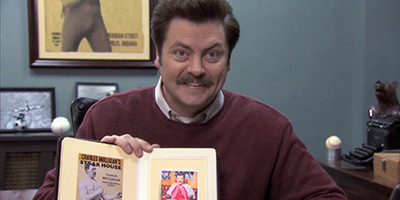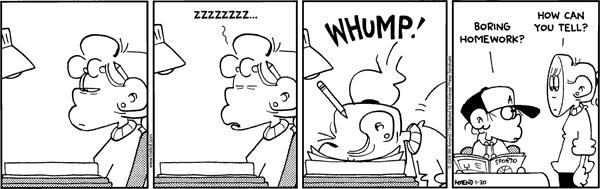Due to the nature of the exercises in this homework, instead of the usual 0 - 1 - 2 grading for each exercise, we will instead utilize 0 - 0.5 - 1 grading for each exercise part.

# Exercise 1

Before it closed, Ron Swanson was a frequent patron of Charles Mulligan’s Steakhouse in Indianapolis, Indiana. Ron enjoyed the experience so much, during each visit he took a picture with his steak.Ron also weighed each steak he consumed. He has a record of eating six “22 ounce” Charles Mulligan’s porterhouse steaks. Ron found that these six steaks weighed

$22.4 \text{ oz}, \ 20.8 \text{ oz}, \ 21.6 \text{ oz}, \ 20.2 \text{ oz}, \ 21.4 \text{ oz}, \ 22.0 \text{ oz}$

Suppose that the weight of “22 ounce” Charles Mulligan’s porterhouse steaks follow a $$N(\mu, \sigma^2)$$ distribution and that Ron’s six steaks were a random sample.

(a) Compute the sample standard deviation, $$s$$, of these six steaks.

(b) Construct a $$95\%$$ two-sided confidence interval for the true mean weight of a “22 ounce” Charles Mulligan’s porterhouse steak, $$\mu$$.

(c) Construct a $$95\%$$ confidence lower bound for the true mean weight of a “22 ounce” Charles Mulligan’s porterhouse steak, $$\mu$$.

(d) Construct a $$90\%$$ two-sided confidence interval for the true standard deviation of the weight of a “22 ounce” Charles Mulligan’s porterhouse steak, $$\sigma$$.

# Exercise 2

In 2017, ballots in Champaign-Urbana contained the following question to assess public opinion on an issue:

“Should the State of Illinois legalize and regulate the sale and use of marijuana in a similar fashion as the State of Colorado?”

Suppose that we would like to understand Champaign-Urbana’s 2017 opinion on marijuana legalization. To satisfy our curiosity, we obtain a random sample of 120 Champaign-Urbanians and find that 87 support marijuana legalization.

(a) Construct a $$99\%$$ confidence interval for $$p$$, the true proportion of Champaign-Urbanians that support recreational marijuana legalization.

(b) Suppose that a pollster wants to estimate the true proportion of Champaign-Urbanians that support recreational marijuana legalization to within 0.04, with $$95\%$$ confidence. How many Champaign-Urbanians should this pollster poll? Assume the pollster has no prior knowledge about the proportion.

(c) Now assume the pollster believes that support for legalization is somewhere between $$65\%$$ and $$85\%$$ and they would like to estimate the true proportion of Champaign-Urbanians that support recreational marijuana legalization to within 0.04, with $$90\%$$ confidence. How many Champaign-Urbanians should this pollster poll?

(d) Instead, suppose we obtain a random sample of 80 Champaign voters, of which 55 support recreational marijuana legalization. We also obtain a random sample of 100 Urbana voters, of which 75 support recreational recreational marijuana legalization. Let $$p_C$$ be the true proportion of Champaign voters who support recreational marijuana legalization and let $$p_U$$ be the true proportion of Urbana voters who support recreational marijuana legalization. Calculate a 99% confidence interval for $$p_U - p_C$$.

# Exercise 3

Suppose students in a Statistics class are interested in the average score of an exam, but the instructor has only graded (a random sample of) 13 of the (many) exams. The instructor states that a $$90\%$$ confidence interval for the true mean is given by $$(79.14, 82.86)$$ and that you can assume the grades follow a normal distribution.

Using only this information, calculate $$\bar{x}$$, $$s$$, and finally, a $$95\%$$ confidence interval for $$\mu$$, the true mean of the exam.

# Exercise 4

Professor Professorson, a researcher at Greendale Community College, is interested in the effect of caffeine on the typing speed of students. Professorson obtains a random sample of 8 students who are given 400 mg of caffeine then given a typing test. (Don’t try this at home.) They type an average of 51.4 words per minute (wpm), with a sample standard deviation of 12.3 wpm. He also obtains a random sample of 13 students who are given a placebo before the typing test. The placebo group types an average of 43.9 wpm, with a sample standard deviation of 15.1 wpm. Assume typing speeds follow a normal distribution in both groups.

Construct a 99% confidence interval for $$\mu_C - \mu_P$$, the true difference in average typing speed between the caffeine and placebo groups. Assume that the two population variances are equal.